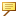Example: Graph Evaluation Function
The graph evaluation function enables you to use graph features to drive dimensions through relations. The dimensions can be section, part, or assembly dimensions. The format is:
evalgraph("graph_name", x)
where
graph_name is the name of a graph.
x is the value along the x-axis of the graph for which the y value is returned.
The next figure shows a sample graph.Graph name: stretch
d1=0.5
d2=1.5
d1=evalgraph ("stretch", 1)
d2=evalgraph ("stretch", d1*4)For sweep features, you can specify the trajectory parameter trajpar as the second argument of this function.